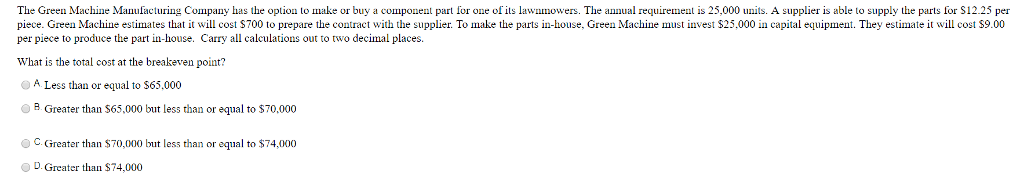# Question & Answer: The Green Machine Manufacturing Company has the option to make or buy a component…..The Green Machine Manufacturing Company has the option to make or buy a component part or one of its lawnmowers. The annual requirement is 25,000 units. A supplier is able to supply the parts for \$12.25 per piece. Green Machine estimates that it will cost \$700 to prepare the contract with the supplier. To make the parts in-house, Green Machine must invest \$25,000 in capital equipment. They estimate it will cost \$9.00 per piece to produce the part in-house. Carry all calculations out to two decimal places. What is the total cost at the breakeven point? A. Less than or equal to S65,000 B. Greater than \$65,000 but less than or equal to \$70,000 C. Greater than \$70,000 but less than or equal to \$74,000 D. Greater than \$74,000

Breakeven is the point where cost to make or buy is same.

Don't use plagiarized sources. Get Your Custom Essay on
Question & Answer: The Green Machine Manufacturing Company has the option to make or buy a component…..
GET AN ESSAY WRITTEN FOR YOU FROM AS LOW AS \$13/PAGE

Let quantity be x at breakeven

Cost to make = Capital cost + Cost per unit for inhouse*x = 25000 + 9x

Cost to buy = Contract cost + Cost per unit by supplier*x = 700 + 12.25x

At breakeven, 25000 + 9x = 700 + 12.25x

24300 = 3.25x

x = 7476.92 units

Total cost at breakeven is = 25000 + 9*7476.92 = 92292.31

Ans: D